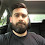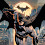# Determination of the moment of inertia of a fly-wheel about its axis of rotation by Falling Weight Method

\${tocify}

## Abstract

In this experiment, the moment of inertia of flywheel is being studied by variating the point of mass of flywheel. The experiment is conducted by recording the time taken by a fixed load until the point where the load is escaping from the flywheel and the number of rotations done after be independent from the load. The moment of inertia then is calculated by substituting the data obtained from the experiment and the experimental value is calculate and compared to the experimental one.

## Introduction

The mass moment of inertia is an important concept in rotational motion. The mass moment of inertia also called as the rotational inertia of a body is a measure of how hard it is to get it rotating about some axis. Rotational inertia is one indicator of the ability of rotating body to store kinetic energy. It is also an indicator of the amount of torque that will be needed to rotational accelerate the body. Just as the mass is a measure of resistance of linear acceleration, moment of inertia is a measure of resistance to angular acceleration.
Flywheel is a solid disc of significant size and weight mounted on the shaft of machines such as steam engines, diesel engines, turbine etc. Its function is to minimize the speed fluctuations that takes place when load on such machines suddenly decreases or increases. The flywheel acquires excess kinetic energy from the machines when load on the machine is less or its running idle and supplies the stored energy to the machine when it is subjected to larger loads. The capacity of storing / shedding of kinetic energy depend on the rotational inertia of the flywheel. This rotational inertia is known as moment of inertia of rotating object namely wheels.
Fig: Hanging Flywheel

## Theory

The rotational kinetic energy can be defined by the equation K = 1/2 mv²
Where, I is the moment of inertia of the body about the axis of rotation.

In this experiment, the flywheel rotates freely about a horizontal axis. The radius of the axle of the flywheel can be measured with a caliper. As m falls, its gravitational potential energy is transferred into translational kinetic energy of m, rotational kinetic energy of the flywheel and work done by friction. As the flywheel completes N further turns, its original rotational kinetic energy is transferred into friction loss. Assume the flywheel decelerates uniformly. Thus, the moment of inertia of the flywheel can be determined.

The working formula are,
Where,
M = Mass
I = Axis of Rotation
n = Distance
ω = Maximum angular velocity
F = Amount of the work
n1 = Number of revolution
ωa = Average angular velocity
Fig: Experimental setup

## Apparatus

1. Flywheel
2. Weights
3. Cord
4. Stop-watch
5. Set-square
6. Meter scale
7. Slide calipers

## Experimental Data

### Table 1: Data for determining the flywheel radius.

 No. of obs. M.S  reading V.S  reading Total for the diameter = D (cm) Radius D/2  (cm) Mean  Radius (cm) 1 2.4 16 2.48 1.24 1.24 2 2.4 16 2.48 1.24 3 2.4 16 2.48 1.24

### Table 2: Data for determining I

 No . of obj Mas s m  (gm) Height No. of  revolution Mean n1 No. of  revolu tion Mean n2 Time t Mean time    t I Mean  I 1 500 148 17 15.33 43 42.33 17 18.33 1764.407 200360.636 14 42 18 15 42 20 2 600 151 15 16.33 53 50.66 25 22.66 516234.6552 17 51 21 17 48 22 3 700 149 17 17 58 58 24 23 485613.3442 17 58 22 17 58 23

## Result

The moment of inertia of the flywheel measured about its axis of rotation 200360.636 gm-cm2
with an error of 20%.

## Discussion

From the comparison of the theoretical and experimental value of moment of inertia for the flywheel in table 2, this experiment resulted in a very large percentage of error about 20%. This error might be caused from the human error in which this experiment involved almost everything was handled by human measurement instead of machine. Thus, we can detect some source of error such as the miscalculated number of rotations due to the rotation is too many and too long to be calculated. The wheel also rotates at high angular velocity. Thus, person who calculated it might be confused and miscalculation was occurred. There also might be error in recording the time taken in which it was from human mistake whether too immediate or too late to start the recording or while stopping the stopwatch and also it is depending on what type or scale of the clock being used since in this experiment, we have no specific stopwatch to be used, instead, we used our own watch. Since the experimental calculation is not in ideal case, thus, the rest assumption of source of error is pointed on the apparatus itself whether there has the lost of energy to the surrounding. Energy is lost due to frictional force in which the friction between the flywheel with the core and the cord will cause a small fraction of energy loss in heat.
Thus, due to this error, a higher step of precaution should be taken to minimize the error. In this experiment, we done thrice reading on three parts of the experiment while the other one, we did twice repetition. This repetition is a way to minimize the error. However, it could be effective if the one who calculate the rotation is a machine since this will avoid a bit human error. Other than that, we may apply lubricant on flywheel to reduce the friction.

## Conclusion

From the experiment, the moment of inertia of flywheel had been studied in which results in the dependency of mass and radius of the wheel as stated in the equation I = ½ MR^2. From here, as the mass and the radius of then flywheel is increased, the moment of inertia is also increased. However, in the comparison of experimental value to the theoretical value, t is found that there are a huge deviation from the theoretical one. Here we conclude the error was done by human mistakes and also might be because of the energy loss due to frictional force. Thus, it is incomparable with the theoretical one because of this is not an ideal condition.

## References

 http://www.123helpme.com/view.asp?id=149828
 Practical physics, Dr. Giasuddin ahmed and Md. Shahabuddin, page- 74
 https://blog.oureducation.in/moment-of-inertia-of-a-flywheel-by-falling-weight-method/
http://www.markedbyteachers.com/gcse/science/measuring-the-moment-of-inertia-of-aflywheel.html

1.From where you find the standard result.

1.The standard result was provided by our lab Teacher after the experiment. Then we have checked our error percentage.

2.Oh. Thanks

2.